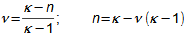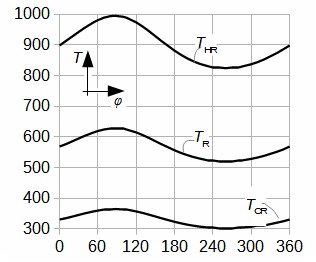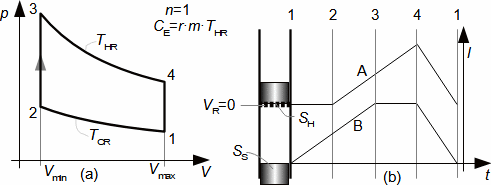﻿ Stirling engine cycle

# 34. Stirling engine cycle

Author: Jiří Škorpík, skorpik.jiri@email.cz

Inside a Stirling engine is transformed heat to work through a closed heat cycle. From a design of heat cycle in p-V diagram can be approximately calculated an internal work of the engine, torque and others engine parameters. An accuracy of these computations is directly proportional to a similarity of shape of the designed cycle to real Stirling engine cycle. The Stirling engine cycle, which is described here is based on several types of heat cycles used to calculation of the Stirling engine cycle, because these cycles have common features (so called assumptions of solving).

## Pressure inside Stirling engine

Pressure of a working gas inside working volume of the Stirling engine is changed under a change of temperature and a volume of the working volume. From description of the Stirling engine can be the working volume separated to three volumes, the death volume, the cylinder volume on the hot side engine and the cylinder volume on the cold side engine of the engine. The mean working gas temperature in all volumes varies during one cycle between their maximum value and their minimum value:1.436 Temperatures of working gas
T [K] temperature; V [m3] volume. Subscript T is term for the hot side engine, subscript S is term for the cold side of the engine, subscript V is term for a cylinder volume, subscript M is term for the death volume, subscript R is term for regenerator, subscript TR is term for the interface between hot volume of the engine and the regenerator, subscript SR is term for the interface between cold volume of the engine and the regenerator. The derivation equation of the mean working gas temperature inside the regenerator is shown in the Appendix 436 or [2, p. 69].

The calculation of the Stirling engine cycle, which is described here is can use under these simplifying assumptions: (1) Value of mean polytropic index of thermodynamics processes inside working volumes of engine is the same during one cycle. – (2) Temperature ratio at border of regenerator is constant, τ=TTR/TSR=const. – (3) There is no pressure loss, pressure of working gas is same in all working volumes. (4) Working gas is ideal gas. – (5) Stirling engine is perfect sealed. – (6) Stirling engine cycle is steady (the same cycle is repeated).

An equation for working gas pressure inside the engine as function its volume can be derived from the assumptions of solvings, which are presented in a previous paragraph:2.437 The equation of the working gas pressure inside the engine as function of the working volume
p [Pa] pressure of working gas; Cint [Pa·m3] integration constant; n [-] mean value of polytropic index (polytropic index is function of change volume); τ [-] temperature ratio between hot and cold side of regenerator; τR [-] temperature ratio between temperature on hot side of regenerator and mean temperature in regenerator; Vred [m3] reduced volume. Derivation of this equation is shown in the Appendix 437. This equations was first published in , .

Last equation shows that only temperatures on boundaries regenerator influences progress of pressure and not mean temperatures in the cylinders. For running engine is necessary difference of temperature between hot and cold side of the engine.

Physical interpretation of the integration constant can be obtainable through a derivation of pressure equation:3.438 Physical interpretation of CintThe integration constant can be computed from any point of cycle, in which be known pressure and a value of reduced volume.

## Polytropic index and isothermal ratio

The polytropic index inside the Stirling engine may be in the interval <1; κ>; (κ [-] heat capacity ratio – adiabatic index) for steady cycle. The polytropic index can not be less than 1. If n is equal κ, then an engine is perfectly thermally isolated and between the hot and cold side of the engine can not occur temperature difference (τ is equal 1 and internal work of the engine is zero). In case n=1 only isothermal processes are performed in the engine, therefore the isothermal processes can be regarded as comparative processes for real processes:4.446 Definition of mean polytropic indexν [-] isothermal ratio*.
*Isothermal ratio
The isothermal ratio determines how much polytropic process inside the working volume is similar to isothermal process. Its value can be in interval <0; 1>. If value of isothermal ratio is 1, then thermodynamic process inside the working volume is isothermal process. If value of isothermal ratio is 0, then thermodynamic process in the working volume is adiabatic process. The difference κ-1 is the maximum deviation between polytropic process and isothermal process inside the working volume.

The isothermal ratio about 0,5 is the usual value for engines with ideal heat transfer between the working gas and heat flow area (e.g. Strojírny Bohdalice Stirling engines, United Stirling V160). The isothermal ratio smaller than 0,5 is the usual value for engines with small heat flow area, higher speed, small death volumes. The isothermal ratio bigger than 0,5 is the usual value for engines with bigger heat flow area, small speed, bigger death volume or engines with control heat flow (difficult to achieve). Polytropic index is function engine speed, engine geometry and working gas.

Measured of the isothermal ratio can shows constructional deficiencies of the engine.

Equtions for calculation of mass and temperature of the working gas, the internal work of the engine, heat input, heat rejection and regenerated heat during one cycle are shown in article 35. Energy balance of Stirling engine cycle.

## Pressure profile inside Stirling engine with crankshaft

The motion of the piston is often performed through a crankshaft, then the volumes of the engine are function of an angle of rotation φ (VTV(φ); VSV(φ)):5.439 The kinematic of pistons of an α-configuration of the Stirling engine
φ [rad] angle of rotation; α [rad] phase angle of motion of pistons; R [m] length of crank; l [m] length connecting rod; L [m; %] hot piston position T and cold piston position S; S [m2] area cross section of cylinder on hot side T and area cross section of cylinder on cold side S.

In this case for calculation of the pistons position can be used the equation for piston position conencted with crankshaft:6.440 Cylinder volumes as function φ

Combination of Equation 2 with Equation 6 be obtained the equation of pressure as function φ. From extremes of function p(φ) can be calculate minimum, maximum pressure and pressure ratio during one cycle φ<0; 2π):7.442 Maximum and minimum pressure and pressure ratio
ε [-] pressure ratio; φmin [rad] angle of rotation at minimum reduced volume Vred,min; φmax [rad] angle rotation at maximum reduced volume Vred,max. If pmax is known; Cint can be calculated from equation for pmax. The angles φmin; φmax can be computed by iteration from Equation (a) and for first step is used Equation (b) and (c) (φmin; φmax for case sinus motion of pistons). Derivation these equations is shown in the Appendix 442.

The mean pressure of cycle be computed according to the mean value theorem which is applied on function p(φ):8.443 Mean pressure of cycle

If the enter of calculation contains the mean pressure of cycle then Cint can be determined through iteration from result Equation 8.

Through same procedure can be derived of equations of piston motion for others configurations of the Stirling engine.

An α-configuration of the Stirling engine which is filled by helium, with crankshaft and about this parameters: cylinder diameter 68 mm (on hot side and cold side are the same diameter), lenght of crank 22 mm, length connecting rod 105 mm, death volume on hot side 110 cm3, death volume on cold side 90 cm3, the regenerator volume 68,682 cm3, the mean temperature of the working gas on hot side of the regenerator 900 K, the mean temperature of the working gas on cold side of regenerator 330 K, the mean pressure 15 MPa, the phase angle 105°. Find progress of pressure as function angle of rotation and others significant angles.
Problem 1.444Problem 1: pressure profile inside engine.

## Temperature change of working gas inside Stirling engine

If n≠1 then temperature of the working gas is changed inside individual volumes according equations:9.251 Temperature of the working gas inside individual volumes of the Stirling engine
TT [K] temperature of working gas on hot side engine; TS [K] temperature of working gas on cold side engine; TR [K] temperature of working gas in regenerator. These equations was derived by these simplifying assumptions TTR=TT; TSR=TS; subscript st is term mean temperature of the working gas during one cycle. Derivation of these equations is shown in the Appendix  251. This equations was first published in .

From these equations is evident, that temperature change follows pressure change and this change is the bigger the bigger is the mean temperature of the working gas in the individual volume. If mean temperature of the working gas is known only, then for a calculation of the temperature TT,max be must used iteration calculation. It means that during first step of the calculation be must estimate of TT,max and by the Equation 9 computes the temperature TT,st. This result must be equal with the required mean temperature on hot side the engine.

Find the temperature change of the working gas during one cycle on hot and cold side and in the regenerator of the Stirling engine about parameter from Problem 1.
Problem 2.819Results of Problem 2.

## Stirling cycle and Schmidt cycle

The Stirling cycle and a Schmidt cycle* are simplified Stirling engine cycles with assumtion n=1. This methods are very very popular. The Stirling cycle assumes linear motion of pistons and zero death volumes, the Schmidt cycle assumes sinusoidal move of pistons and non-zero death volumes. Details about these cycles are shown in , .

Gustav Schmidt; 1826-1881
Professor at German Polytechnic in Prague. He published his cycle at 1871.10.447 Stirling cycle
(a) p-V diagram; (b) trajectory of pistons. r [J·kg-1·K-1] individual gas constant of working gas; m [kg] mass of working gas inside working volume; t [s] time. A trajectory of hot piston; B trajectory of cold piston. Derivation this situation is shown in the Appendix 447.

## Other comparative cycles for Stirling engine

Theodor Finkelstein be published cycle where n=κ, this cycle be computed by Finite element methods , [2, p. 87]. The most widely is cycle with adiabatic processes in cylinders and isothermal processes in death volumes. Authors this cycle are Israel Urieli and David Berchowitz  (authors assembled set of differential equations, which are solved by Runge – Kuttak method).

## Words on conclusion

Thermodynamic cycle described here is based on mean values of temperature ratio and polytropic index, but these quantities are variable during one cycle at real process. Mass of the working gas in the working volume is not constant also at real process (alternate ingress/leakage of the working gas through piston rings, see article 36. Losses in Stirling engines). These factors (especially the last said) significantly influence calculated diagrams.

## References

1. URIELI, Israel, BERCHOWITZ, David. Stirling Cycle Engine Analysis, 1984. 1. vydání. Bristol: Adam Hilger Ltd., ISBN 978-0996002196.

2. MARTINI, William. Stirling engine design manual, 2004. Přetisk vydání z roku 1983. Honolulu: University press of the Pacific, ISBN: 1-4102-1604-7.

3. WALKER, Graham. Dvigateli Stirlinga/Двигатели Стирлинга, 1985. Doplněný Ruský překlad knihy: WALKER, Graham Stirling engine, 1980. Oxford: Oxford University Press.

4. ŠKORPÍK, Jiří. Příspěvek k návrhu Stirlingova motoru, VUT v Brně, Edice PhD Thesis, 2008, ISBN 978-80-214-3763-0.

5. ŠKORPÍK, Jiří. A new comparative cycle of a Stirling engine, The 14th International Stirling Engine Conference, in Groningen – Netherlands, 2009, ISBN: 978-88-8326-022-3.

6. ŠKORPÍK, Jiří. Stirling engine cycle-supplement, The 15th International Stirling Engine Conference, in Dubrovnik-Croatia, 2012, ISBN: 978-88-8326-019-3.